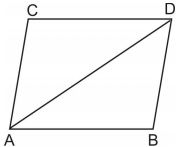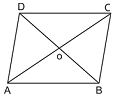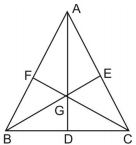# Areas of Parallelograms and Triangles class 9 Notes Mathematics## myCBSEguide App

CBSE, NCERT, JEE Main, NEET-UG, NDA, Exam Papers, Question Bank, NCERT Solutions, Exemplars, Revision Notes, Free Videos, MCQ Tests & more.

CBSE class 9 Mathematics Chapter 9 Areas of Parallelograms and Triangles notes in PDF are available for free download in myCBSEguide mobile app. The best app for CBSE students now provides Areas of Parallelograms and Triangles class 9 Notes latest chapter wise notes for quick preparation of CBSE board exams and school based annual examinations. Class 9 Mathematics notes on Chapter 9 Areas of Parallelograms and Triangles are also available for download in CBSE Guide website.

## CBSE Guide Areas of Parallelograms and Triangles class 9 Notes

CBSE guide notes are the comprehensive notes which covers the latest syllabus of CBSE and NCERT. It includes all the topics given in NCERT class 9 Mathematics text book. Users can download CBSE guide quick revision notes from myCBSEguide mobile app and my CBSE guide website.

## 9 Mathematics notes Chapter 9 Areas of Parallelograms and Triangles

Download CBSE class 9th revision notes for Chapter 9 Areas of Parallelograms and Triangles in PDF format for free. Download revision notes for Areas of Parallelograms and Triangles class 9 Notes and score high in exams. These are the Areas of Parallelograms and Triangles class 9 Notes prepared by team of expert teachers. The revision notes help you revise the whole chapter in minutes. Revising notes in exam days is on of the best tips recommended by teachers during exam days.

CBSE Class 09 Mathematics
Revision Notes
CHAPTER 9
AREAS OF PARALLELOGRAMS AND TRIANGLES

1. Figures on the same Base and between the same Parallels

2. Parallelograms on the same Base and between the same Parallels

3. Triangles on the same Base and between the same Parallels

• Area of a parallelogram = base height
• Area of a triangle =  base height
• Area of a trapezium = (sum of parallel sides) distance between them
• Area of rhombus =  product of diagonals
• Two figures are said to be on the same base and between the same parallels, if they have a common side (base) and the vertices (or the vertex) opposite to the common base of each figure lie on a line parallel to the base.
• Parallelogram on the same base and between the same parallels are equal in area.
• If each diagonal of a quadrilateral separates it into two triangles of equal area, then the quadrilateral is a parallelogram.
• A parallelogram and a rectangle on the same base and between the same parallels are equal in area.
• Triangles on the same base and between the same parallels are equal in area.
• Triangles having equal areas and having one side of one of the triangles, equal to one side of the other, have their corresponding altitude equal.
• If a triangle and parallelogram are on the same base and between the same parallels, then.

Area of triangle = x area of the parallelogram

• A diagonal of parallelogram divides it into two triangles of equal areas.

In parallelogram ABCD, we have

Area of• The diagonals of a parallelogram divide it into four triangles of equal areas therefore

===• A median AD of a  divides it into two triangles of equal areas. Therefore
• If the medians of a intersect at G, then

===## Areas of Parallelograms and Triangles class 9 Notes

• CBSE Revision notes for Class 9 Mathematics PDF
• CBSE Revision notes Class 9 Mathematics – CBSE
• CBSE Revisions notes and Key Points Class 9 Mathematics
• Summary of the NCERT books all chapters in Mathematics class 9
• Short notes for CBSE class 9th Mathematics
• Key notes and chapter summary of Mathematics class 9
• Quick revision notes for CBSE board exams

## CBSE Class-9 Revision Notes and Key Points

Areas of Parallelograms and Triangles class 9 Notes. CBSE quick revision note for Class-9 Mathematics, Chemistry, Maths, Biology and other subject are very helpful to revise the whole syllabus during exam days. The revision notes covers all important formulas and concepts given in the chapter. Even if you wish to have an overview of a chapter, quick revision notes are here to do if for you. These notes will certainly save your time during stressful exam days.

To download Areas of Parallelograms and Triangles class 9 Notes, sample paper for class 9 Mathematics, Social Science, Science, English Communicative; do check myCBSEguide app or website. myCBSEguide provides sample papers with solution, test papers for chapter-wise practice, NCERT solutions, NCERT Exemplar solutions, quick revision notes for ready reference, CBSE guess papers and CBSE important question papers. Sample Paper all are made available through the best app for CBSE students and myCBSEguide website.### 1 thought on “Areas of Parallelograms and Triangles class 9 Notes Mathematics”

1. According to me you should explain it deeply and with more examples so students can understand properly ..and thanks.